### Home > CAAC > Chapter 4 > Lesson 4.2.1 > Problem4-72

4-72.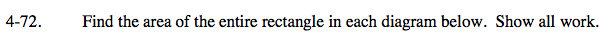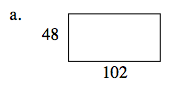Area = (base)(height)

Area = (48)(102)

Area = 4896 sq. units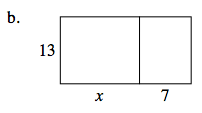Area = (base)(height)

Separate the rectangle into two rectangles, then add the two areas to find the total area.

Area = (13· x) + (13· 7)

Area = 13x + 91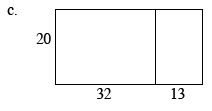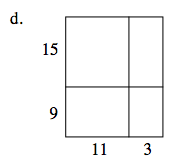For parts (c) and (d), use the same strategy outlined in part (b).